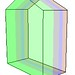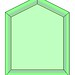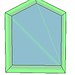# Polygon Triangulation And Layer Filling

Hi! I’m new here. Just started working with OpenGL using MFC. I’ve run into a problem when filling a polygons triangulated areas with different layers.

1st… I have a simple polygon (5 points in this example). I triangulate that polygon using googled methods to form 3 different triangles to fill. The polygon is in 3D and is a few layers thick.When I fill the top layer transparently everything looks smooth,but when filling any layer below it and moving the camera around in 3D space, I see the triangle lines between the layers.My code is as follows:
glEnable(GL_BLEND);
glDisable(GL_DEPTH_TEST);
glBlendFunc(GL_SRC_ALPHA, GL_ONE_MINUS_SRC_ALPHA);
glPolygonMode(GL_FRONT_AND_BACK, GL_FILL);
glLineWidth(1);
////////removed code: here I draw the side quads for the 3d polygon
glBegin(GL_TRIANGLES);
for (j=0;j<tcount;j++){
const Vector2d &p1 = triangulationresult[j3+0];
const Vector2d &p2 = triangulationresult[j
3+1];
const Vector2d &p3 = triangulationresult[j*3+2];
glVertex3f(p1.GetX(),p1.GetY(), zValueForTopLayer);
glVertex3f(p2.GetX(),p2.GetY(), zValueForTopLayer);
glVertex3f(p3.GetX(),p3.GetY(), zValueForTopLayer);
//if i add this code here, i see the lines for the triangles
//glVertex3f(p1.GetX(),p1.GetY(),zValueForBottomLayer);
//glVertex3f(p2.GetX(),p2.GetY(), zValueForBottomLayer);
//glVertex3f(p3.GetX(),p3.GetY(), zValueForBottomLayer);
}
glEnd();

How do I fix??

There must be some kind of setting… a blend function or something… that smooths those triangle faces to become one face for the polygon?

Here’s some other settings I have in the openGL Init:

// Set color to use when clearing the background.
glClearColor(1.0f, 1.0f, 1.0f, 1.0f);
glClearDepth(1.0f);

// Turn on backface culling
glFrontFace(GL_CCW);
glCullFace(GL_BACK);

// Turn on depth testing
glEnable(GL_DEPTH_TEST);
glDepthFunc(GL_LEQUAL);

Make sure GL_POLYGON_SMOOTH is disabled.

Still seeing the trianglation in the polygon with GL_POLYGON_SMOOTH disabled. (for reference I am drawing triangles, not polygons). I decided to go another route to graphically display the information I want (a solid color throughout), but for future projects I am still interested in a solution for this.

How are you ensuring that the vertex positions are binary-identical?

I’m not even sure what you mean by binary-identical, but if you mean that they are the same numbers for a shared point of 2 triangles, the only verification I have is visual studio displaying them the same up to a certain decimal precision (5 points). These are floats. I tried casting all vertex coordinates into integers, and came up with the same result.

Is that what you meant?

Have to ask - why do U triangulate the original polygon?
Why not use glBegin(GL_POLYGON) instead of glBegin(GL_TRIANGLES)?
That would be the first change I’d suggest.

Binary identical. As in “float1 == float2” is true.

GL_POLYGON won’t draw concave polygons.

Is there any way your triangulation routine is working inconsistently on different layers? If you draw all the polys in wireframe, does anything look funny? Also, if you draw the bottom layer without drawing the top layer, does it look o.k.?

What I’m doing is:

1. Getting the triangulation vertices for the polygon.
2. Displaying them using GL_TRIANGLES in one color
3. Moving down the Z axis and displaying the next layer with the exact same x/y numbers, but in a different color

Drawing the bottom layer alone displays perfectly fine.
Drawing the top layer alone displays perfectly fine.
Drawing the top and bottom layer of both the same color displays perfectly fine.

The problem I run into is displaying the top and bottom layer as different colors (this is when I see lines).

Don’t know what to tell you. I’m not having any trouble doing what you’re trying to do. I wrote a little program that displays 3 layers of house-shaped, transparent, polys. It works fine. The pic below shows wireframe and filled versions. I can rotate it to any orientation and the transparency seems to work correctly. I don’t get the lines you get. I didn’t use your code exactly, but my code is very similar. I’ve included the pertinent parts of it. If you have time, there are 2 experiments you could try: 1) run your code on another computer (maybe you have driver problems), 2) test to see if the problem is numerical (as suggested by another poster), enter coordinates of the vertices explicitly rather than computing them on the fly.``````//---+----3----+----2----+----1----+---<>---+----1----+----2----+----3----+----4
//--------------------------------   Houses   ----------------------------------

void Houses (void)
{
short i;
float z = -0.4;

glBegin (GL_TRIANGLES);

for (i = 0; i < 3; i++)  {
z += 0.4;
switch (i)  {
case 0:  glColor4f (0.8, 0.4, 0.1, 0.5);  break;
case 1:  glColor4f (0.1, 1.0, 0.5, 0.5);  break;
case 2:  glColor4f (0.1, 0.5, 1.0, 0.5);  break;
}

glVertex3f (0,0,z);  glVertex3f (0.5,1.5,z);  glVertex3f (0.0,1.0,z);
glVertex3f (0,0,z);  glVertex3f (1.0,1.0,z);  glVertex3f (0.5,1.5,z);
glVertex3f (0,0,z);  glVertex3f (1.0,0.0,z);  glVertex3f (1.0,1.0,z);
}

glEnd ();
}

//---+----3----+----2----+----1----+---<>---+----1----+----2----+----3----+----4
//--------------------------------   Layers   ----------------------------------

void Layers (void)
{
glEnable      (GL_BLEND);
//  glEnable      (GL_CULL_FACE);
glDisable     (GL_DEPTH_TEST);
glBlendFunc   (GL_SRC_ALPHA, GL_ONE_MINUS_SRC_ALPHA);
//  glPolygonMode (GL_FRONT_AND_BACK, GL_LINE);
glPolygonMode (GL_FRONT_AND_BACK, GL_FILL);

Houses ();
}

//---+----3----+----2----+----1----+---<>---+----1----+----2----+----3----+----4
//-------------------------------   Display   ----------------------------------

void Display (void)
{
glClear (GL_COLOR_BUFFER_BIT | GL_DEPTH_BUFFER_BIT);

glMatrixMode (GL_PROJECTION);
gluPerspective (40.0, 1.5, 0.1, 10.0);

glMatrixMode (GL_MODELVIEW);

glTranslatef (-0.5, -0.6, -5.0);

glRotatef (tippangle, 1,0,0);  // Up and down arrow keys 'tip' view.
glRotatef (viewangle, 0,1,0);  // Right/left arrow keys 'turn' view.

Layers ();

glutSwapBuffers();
}

//---+----3----+----2----+----1----+---<>---+----1----+----2----+----3----+----4

int main (int argc, char **argv)
{
glutInit               (&argc, argv);
glutInitDisplayMode    (GLUT_DOUBLE | GLUT_RGB);
glutInitWindowPosition (300, 200 );
glutInitWindowSize     (600, 400 );
glutCreateWindow       ("Multiple Layer Transparency");

glClearColor (0.2, 0.2, 0.2, 0.0);

glutDisplayFunc (Display);
glutReshapeFunc (ReSize);
glutSpecialFunc (Special_Keys);

glutMainLoop ();

return 1;
}

//---+----3----+----2----+----1----+---<>---+----1----+----2----+----3----+----4

``````

Ok, fixed it. Thank you all for your help, especially MaxH for the work put in. Going through your code, I realized that you were drawing all triangles of the polygon, before moving to the next Z coordinate and changing the color.

``````
float z;
glBegin (GL_TRIANGLES);
int j;
for (j=0;j<3;j++){
z = 0;
for (i = 0; i < 3; i++)  {
z += 0.4;
switch (i)  {
case 0:  glColor4f (0.8, 0.4, 0.1, 0.5);  break;
case 1:  glColor4f (0.1, 1.0, 0.5, 0.5);  break;
case 2:  glColor4f (0.1, 0.5, 1.0, 0.5);  break;
}
if (j==0) {glVertex3f (0,0,z);  glVertex3f (0.5,1.5,z);  glVertex3f (0.0,1.0,z);}
else if (j==1) {glVertex3f (0,0,z);  glVertex3f (1.0,1.0,z);  glVertex3f (0.5,1.5,z);}
else if (j==2) {glVertex3f (0,0,z);  glVertex3f (1.0,0.0,z);  glVertex3f (1.0,1.0,z);}
}
}
glEnd ();

``````

…produced…What I was doing wrong before was drawing one triangle of the polygon, changing color and z coordinate to draw next layer… then going to next triangle of polygon with original z and color, and repeating.

I’m not really sure why this made a difference, but changing it around to drawing all triangles of one color and Z first, then switching color and z coordinate and drawing all triangles again works just fine.

For anyone who has same problem, check the order of your loops, and always draw all items of one color on one layer at a time, before moving to the next layer.